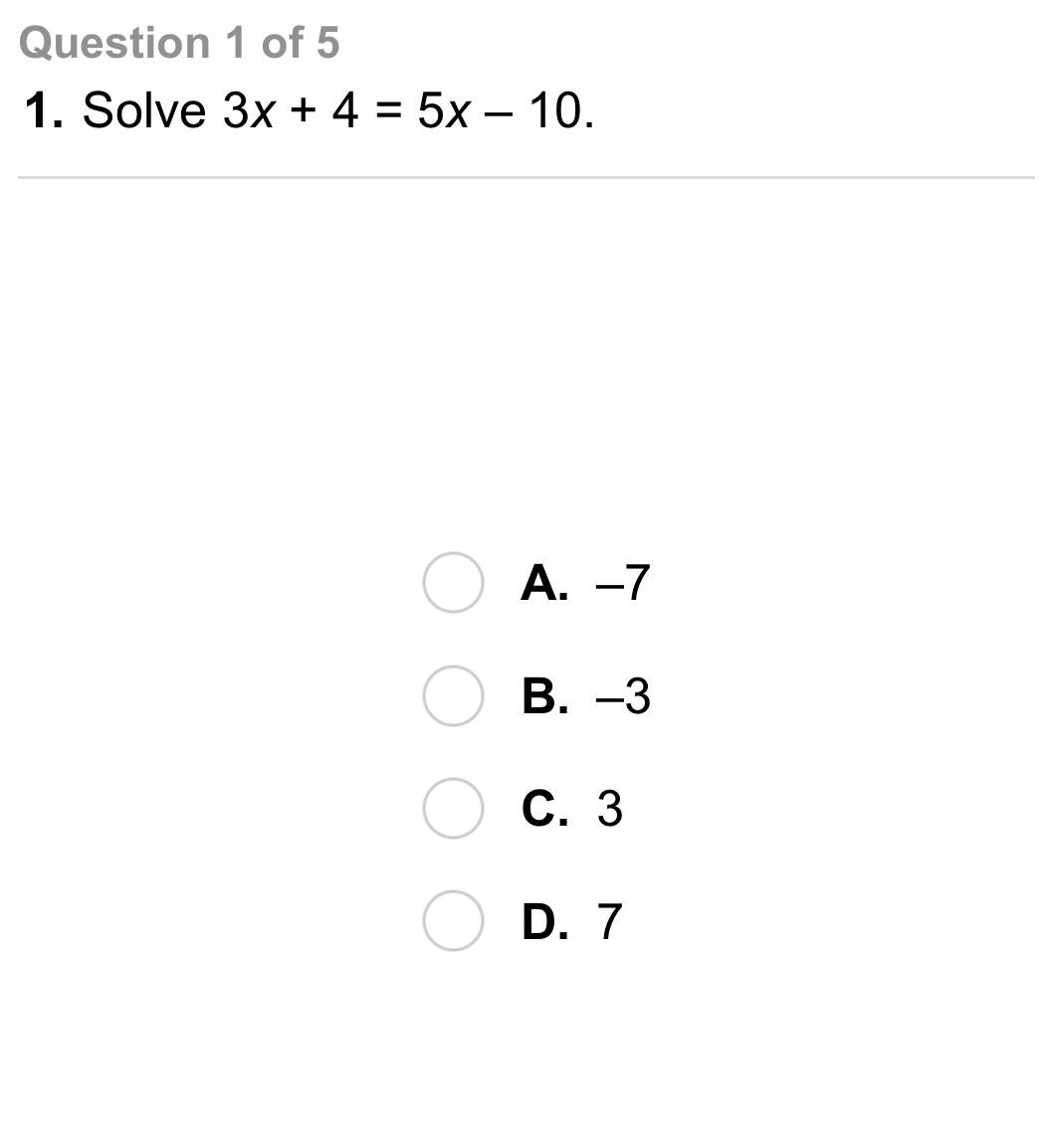Algebra 1 2-4 Independent Practice: Solving Equations with Variables on Both Sides
starstarstarstarstarstarstarstarstarstar
by Matthew Richardson
| 10 Questions1
1
10
A
B
C
D2
2
10
A
B
C
D3
3
10
A
B
C
D4
4
10
A
B
C
D5
5
10
A
B
C
D6
10
Vocabulary: How many solutions does the equation have?

Infinitely many solutions
One solution
No solutions
7
10
Vocabulary: How many solutions does the equation have?

Infinitely many solutions
One solution
No solutions
8
10
Vocabulary: How many solutions does the equation have?

Infinitely many solutions
One solution
No solutions
9
10
Writing: A student solved an equation and found that the variable was eliminated in the process of solving the equation. How would the student know whether the equation is an identity or an equation with no solution?
10
10
Reflect: Math Success